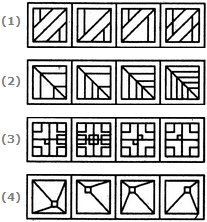# Non Verbal Reasoning - Rule Detection - Discussion

Discussion Forum : Rule Detection - Section 1 (Q.No. 5)
Directions to Solve

In each of the following questions, choose the set of figures which follows the given rule.

5.

Choose the set of figures which follows the given rule.

Rule: The series becomes complex as it proceeds.1
2
3
4
Explanation:
No answer description is available. Let's discuss.
Discussion:
2 comments Page 1 of 1.

Ariel said:   9 years ago
The second one is the correct answer because more and more lines are added in the pattern. The first one first decrease the number of lines, so it is wrong. The third and fourth one also didn't become more complete, they even get simpler. So the correct answer is B.
(1)